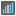• # Code works on native interpreter

## Question related to missionMedian

I would like to give some feedback about ...

HTTP_USER_AGENT:

```Mozilla/5.0 (Macintosh; Intel Mac OS X 10_9_4) AppleWebKit/537.36 (KHTML, like Gecko) Chrome/37.0.2062.120 Safari/537.36
```

My Code:

```def checkio(data):
data.sort()
length = len(data)
median = None
if length % 2:
# Odd len
median = data[int(length/2)]
else:
# Even len
median = (data[(length/2) - 1] + data[length/2])/2

#replace this for solution
return median

#These "asserts" using only for self-checking and not necessary for auto-testing
if __name__ == '__main__':
assert checkio([1, 2, 3, 4, 5]) == 3, "Sorted list"
assert checkio([3, 1, 2, 5, 3]) == 3, "Not sorted list"
assert checkio([1, 300, 2, 200, 1]) == 2, "It's not an average"
assert checkio([3, 6, 20, 99, 10, 15]) == 12.5, "Even length"
print("Start the long test")
assert checkio(list(range(1000000))) == 499999.5, "Long."
print("The local tests are done.")
```